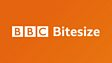An animated guide to probability explains how to express probability as fractions and percentages based on the ratio of the number of ways an outcome can happen and the total number of outcomes. Experimental probability and the importance of basing this on a large trial are also covered.This clip is from:
Bitesize Clips

Use as an introduction or recap to lessons on simple probability or in lessons relating to experiments and trials. Discuss with students what would make a good size of trial for different types of experiment. Should more trials be considered for experiments with more possible outcomes?